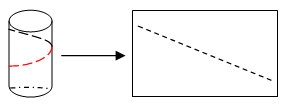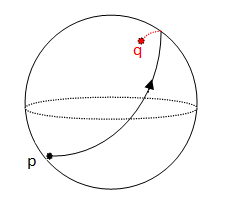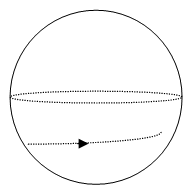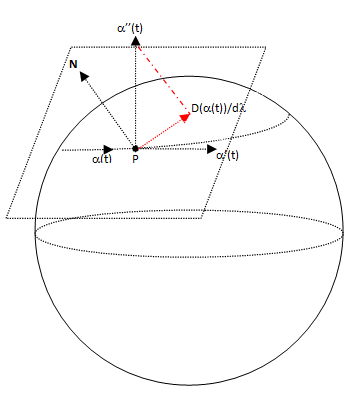# Covariant derivate and Geodesics

### Basic concepts and principlesFig.1 The cylinder and the plane areisometric therefore not subject to forces become one of the otherc
Given a certain manifold, we wonder about trajectories followed by particles non subject to forces.

For example, in the plane case, particles non subjected to forces obviously follow straight trajectories.

If we consider a cylinder, we don't know at first what are these trajectories, but we can infer that are of the form given by Figure 1. because cylinder and plane are isometric manifolds and such trajectories are transformed into straight lines in the plane (or the straight lines are transformed into those paths) by an isomorfism.Fig. 2 If the particle is not subjected to other forces it follow a trajectory given by the maximum circle defined by the starting position and the initial direction
In general, given a curve with its image defined on a differentiable manifold, we assume that it is a particle moving into our differentiable variety. Our goal is to know what is the motion if particle is not subjected to external forces.

Suppose that earth were a perfect sphere with smooth surface and without friction and at some point p one person throws a bowling ball flush

Such ball will travel a flat trajectory (in the sphere viewpoint) and, without friction, the inertia effect due to initial impulse join gravity will continue circling the earth.

In this half trivial example, it is clear that the ball describes trajectories given by the maximum circle defined by the start point and the initial ball throwing direction, because such trajectory passes through the antipodal point to intial point p, call it q, Fig 2.

Therefore we can say that if we see a particle in motion such throught a parallel, it is subject to any external force because it are undergoing an acceleration Fig 3.Fig.3 If a particle moves in the sphere surface throught a trajectory that is not a maxumum circle, we can say that is subject to forces

### Extended Theory

We saw how to define a tensor field in section Tensors and tensorial algebra.
Given then a vector field on a manifold M (recall that a vector is a tensor (1.0)), we can calculate its derivative. For example, suppose the field has components

$V=V^i\frac{\partial }{\partial x} = V^i \partial_i$

The derivative with respect to xj is not $\frac{\partial V^i }{\partial x^j}$, but is (by applying chain rule)

$\frac{\partial V}{\partial x^j} = \frac{\partial V^i}{\partial x^j} \frac{\partial}{\partial x^i} + V^i \frac{\partial^2 }{\partial x^i \partial x^j}$

Note that the term with the second derivative leaves out of tangent space.

However, if we substitute it for

$\Gamma^k _{ij} = \frac{\partial^2 }{\partial x^i \partial x^j} \partial_k$

we have

$\frac{\partial V}{\partial x^j} = (\frac{\partial V^k}{\partial x^j} + \Gamma^k _{ij} V^i) \partial _k$

This is like take projection on tangent space TP(M) of the component in derivate that does not belong to TP(M).

The $\Gamma^k _{ij}$ are called Christoffel Symbols.

What we got so, is the projection ontangent space TP(M) of derivative of the vector field.

This construction is what is called the covariant derivative of a vector field, one more time: the covariant derivative is the projection onto tangent space of derivative of the field, Formally.

### Covariant derivative of a vector field on a differentiable manifold definition

Given a semiriemannian manifolds and a vector field defined on it, denoted by

$V = V^i \partial _i$

It is call covariant derivate of V in a j direction to an one (1, 1) tensor whose components are

$\frac{D V^i}{d x^j} = V_{;j} = (\frac{\partial V^k}{\partial x^j} + \Gamma^k _{ij} V^i) \partial _k$

Thus the covariant derivative at direction xj is the vector

$\nabla _j V = V^i_{;j} \partial _i$

### Notation

It is common in differential geometry, to denote the partial derivative as a comma join a subscript of the form

$\frac{{\partial V}}{{\partial x^i}} = V_,i$

And denote the covariant derivative as a semicolon (;) and a subscript of the form

$\frac{DV}{dx^i} = V_;i$

We will specialize a bit more, consider a trajectory and we take it vector tangent at each point to the path as field vector. This allows us to define the covariant derivative of trajectory as the derivative of this vector field

### Covariant derivative of a trajectory definition

Lets

$\gamma(\lambda) = (x^1(\lambda), ..., x^n(\lambda))$

a differentiable curve with its image in a semiriemannian variety M joining two points p and q of M. V is the vector field formed by the tangent vector of $\gamma)$.

Is called covariant derivative of V along $\gamma)$ to

$\frac{DV}{d\lambda} = (\frac{dV}{d\lambda} + \Gamma_{ij}^k V^{i}\frac{dx^j}{d\lambda}) \partial _k$

If

$\frac{DV}{d\lambda} = 0$

then it is said that V is a parallel transport along $\gamma)$ from p to q.

### Geodesic definition

Lets

$\gamma(\lambda) = (x^1(\lambda), ..., x^n(\lambda))$

a differentiable curve with its image in a semiriemannian variety M joining two points p and q of M. V is the vector field formed by the tangent vector of$\gamma)$.

It says that $\gamma)$ is a geodesic if the full image holds that

$\frac{DV}{d\lambda} = 0$

Or, equivalently

$\frac{d^2x^k}{d\lambda^2} + \Gamma_{ij}^k \frac{dx^i}{d\lambda}\frac{dx^j}{d\lambda} = 0$Fig.4 The covariant derivative of a trajectory is the projection on tangent space TP(M) of trajectory tangent vector α'(t)

A particle not subjected to forces on a manifold moves along trajectories called geodesic

A particle moving along a geodesic is not subjected to forces? this is not correct because the particle may still suffer acceleration by modifying the lenght of tangent vector. The following theorem tells us that a particle non subject to forces moves along a geodesic and tangent vector could not vary its length

### Theorem: conservation of vector tangent length on a geodesic

Lets

$\gamma(\lambda) = (x^1(\lambda), ..., x^n(\lambda))$

a geodesic in a variety semiriemannian M and G is it metric. Then

$G(V(\lambda), V(\lambda))$

Is constant.

# Was useful? want add anything?

Post here

### Post from other users

Post here